# Validate Binary Search Tree

The problem states that we will be given a binary tree and we have to determine if it is a valid binary search tree(BST) or not. you can find this problem in Leetcode and in Hackerrank.

First of all, what is a binary tree?

A binary tree is a tree that has at most two child nodes.

Then what is a binary search tree?

A binary search tree(BST) is a kind of binary tree where each node is greater than its left node and smaller than its right node.

If you do not know how to implement a binary search tree you read my post about it.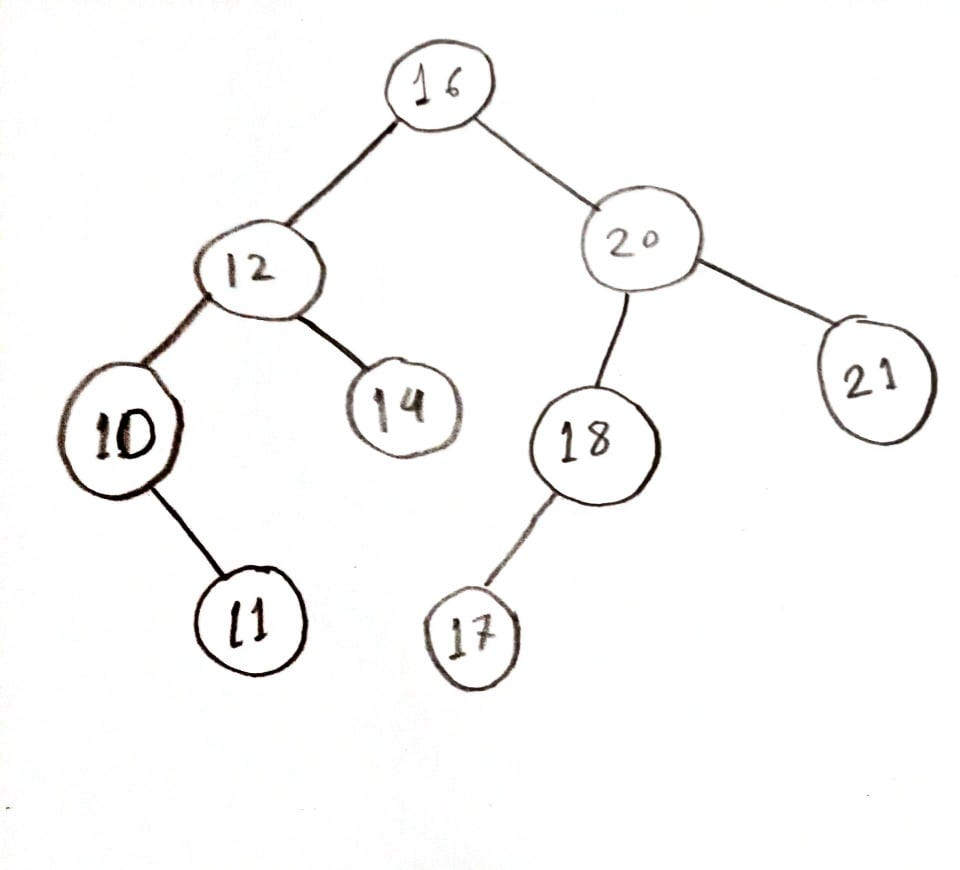Example of a binary search tree(BST)

In the above picture, we have a valid binary search tree(BST). In order to validate a BST, we have to check if the current node is greater than the left node and smaller than the right node. If the whole tree abides by this rule then it is a valid BST, otherwise, it is not.

Code:

How does the code work?

As we will be comparing each node with its left and right node, initially we don’t know the right and left node for the root node so, we are taking the base left value as LONG_MIN and base right value as LONG_MAX. And for each node, we are checking if it matches our BST definition. We go through the code for the binary search tree I have provided in the picture above.

The first-time validateBstHelper(16, -2147483647, 2147483647) function is called, it checks if it null then it checks if it is less than the minimum value or greater than the maximum value. And none of these are true, so it goes to else if and calls validateBstHelper function for the left node validateBstHelper(12, -2147483647, 16) notice how the maximum value is changed, remember the definition current node has to be greater than the left node? that’s why.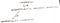recursive call till validateBstHelper(12, -2147483647, 16)

Again for validateBstHelper(12, -2147483647, 16) it will check if it is null then it checks if it is less than the minimum value or greater than the maximum value. None of these are true so it goes to the else if block and call validateBstHelper function for its left node validateBstHelper(10, -2147483647, 12). And again the maximum value changed to the value of the current node.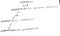recursive call till validateBstHelper(10, -2147483647, 12)

For validateBstHelper(10, -2147483647, 12) it will check if it is null then it checks if it is less than the minimum value or greater than the maximum value. None of these are true so it goes to the else if block and its left node are null so, call validateBstHelper function for its right node validateBstHelper(11, 10, 12). And notice now the minimum value is updated to 10 as the definition says the current node has to be less than the right node.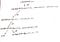recursive call till validateBstHelper(11, 10, 12)

For validateBstHelper(11, 10, 12) it will check if it is null then it checks if it is less than the minimum value or greater than the maximum value. Then it will go to else ifs and see there is no child node of this node, which means both left node and right node are null. So, true will be returned to the method which called this method( i.e. validateBstHelper(10, -2147483647, 12) ).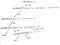recursive call till validateBstHelper(11, 10, 12)

Now we are back to validateBstHelper(10, -2147483647, 12) call and both of its nodes have returned true(if a node is null we are returning true). So, none of the if-else conditions is true for this node and we will return true to the method which called this method( i.e. validateBstHelper(12, -2147483647, 16)).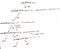Recursive tree after validateBstHelper(10, -2147483647, 12) has returned true

We are back at validateBstHelper(12, -2147483647, 16) and none of the conditions till the left node is true because the left node has already returned true. So, we go to the else if block for its right node validateBstHelper(14, 12, 16).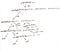recursive call till validateBstHelper(14, 12, 16)

For validateBstHelper(14, 12, 16) there is no child node so, it will return true to its parent node validateBstHelper(12, -2147483647, 16).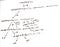Recursive tree after validateBstHelper(14, 12, 16) has returned true

For validateBstHelper(12, -2147483647, 16) both of its child nodes have returned true so, it will return true to its parent node(i.e. validateBstHelper(16, -2147483647, 2147483647)).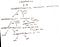Recursive tree after validateBstHelper(12, -2147483647, 16) has returned true

Now for validateBstHelper(16, -2147483647, 2147483647) none of the conditions till its left node will be true because its left node has already returned true. So, it will go to the else if block for the right node validateBstHelper(20, 16, 2147483647).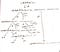Recursive call till validateBstHelper(20, 16, 2147483647)

For validateBstHelper(20, 16, 2147483647) it will check if it is null and if it is less than the minimum value(16) or greater than the maximum value. None of those conditions is true so, it will go to the else if block for its left node validateBstHelper(18, 16, 20).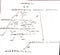Recursive call till validateBstHelper(18, 16, 20)

For validateBstHelper(18, 16, 20) again all the null checks and the check for minimum and maximum will be done. As that condition is false, it will go to the else if block for the left node and validateBstHelper(17, 16, 18) will be called.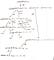Recursive call till validateBstHelper(17, 16, 18)

validateBstHelper(17, 16, 18) it has no child nodes so, it will return true to validateBstHelper(18, 16, 20).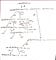Recursive tree after validateBstHelper(17, 16, 18) has returned true

For validateBstHelper(18, 16, 20) none of the conditions till its left node is true because the left node has already returned true. So, now we’ll check if the right node is null or not. But it has no right node, which means this will return true to its parent validateBstHelper(20, 16, 2147483647).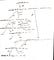Recursive tree after validateBstHelper(18, 16, 20) has returned true

As the left node of validateBstHelper(20, 16, 2147483647) has returned true now method will be called for its right node validateBstHelper(21, 20, 2147483647).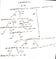Recursive call till validateBstHelper(21, 20, 2147483647)

For validateBstHelper(21, 20, 2147483647) none of the condition is true and it has no child nodes, so it will return true to validateBstHelper(20, 16, 2147483647).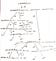Recursive tree after validateBstHelper(21, 20, 2147483647) has returned true

Both child of validateBstHelper(20, 16, 2147483647) has returned true so, it will return true to its parent node validateBstHelper(16, -2147483647, 2147483647). Also both the child of validateBstHelper(16, -2147483647, 2147483647) has also returned true, that means it will return true to isValidBst(16).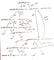Recursive call tree for the whole tree

Complexity

Time complexity is O(n) where n is the number of nodes. It is O(n) because we are traversing every node of the tree.

Space complexity on the average case is O(h) where h is the length of the longest branch of the tree. It is O(h) because we calling the recursive methods and at a time there will be at most h calls in the call stack. Notice the recursive tree above we don’t call right nodes until all its left node has returned and popped from the call stack, similarly, there will be no methods in the call stack for left nodes when we are calling the right node. So, it is O(h) on the average case.

But for worst-case space complexity is O(n) if the tree is like below:

In this case, space complexity is O(n) because the length of the longest branch is the number of nodes.

Software Engineer at LogMeIn